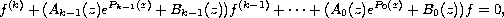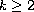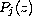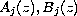Electron. J. Diff. Equ., Vol. 2010(2010), No. 65, pp. 1-12.

### Growth of solutions of higher-order linear differential equations Karima Hamani

Abstract:
In this article, we study the growth of solutions of the linear differential equationwhereis an integer,are nonconstant polynomials andare entire functions, not identically zero. We determine the hyper-order of these solutions, under certain conditions.

Submitted February 3, 2010. Published May 8, 2010.
Math Subject Classifications: 34A20, 30D35.
Key Words: Linear differential equation; entire function; hyper-order.

Show me the PDF file (256 KB), TEX file, and other files for this article.Karima Hamani Department of Mathematics Laboratory of Pure and Applied Mathematics University of Mostaganem, B. P. 227 Mostaganem, Algeria email: hamanikarima@yahoo.fr